Author: Oscar Cronquist Article last updated on July 23, 2022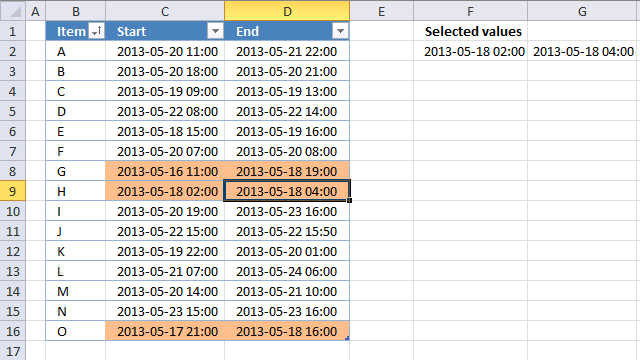This article demonstrates event code combined with Conditional Formatting that highlights overlapping date ranges based on the selected date range.

This method will automatically highlight the selected date range and all other overlapping date ranges, press with left mouse button on any another date range to highlight it and it's overlapping date ranges as well.

Event code is basically a VBA macro that is run when an event is triggered, it can be a specific worksheet being activated or a cell being selected among many other things.

The image above shows cell D9 selected, the event code then copies the date range in cell range C9:D9 to cell range F2:G2. A conditional formatting formula uses the values in cells F2 and G2 to highlight other date ranges in the Excel Table that overlaps.

## 1. Create an Excel Table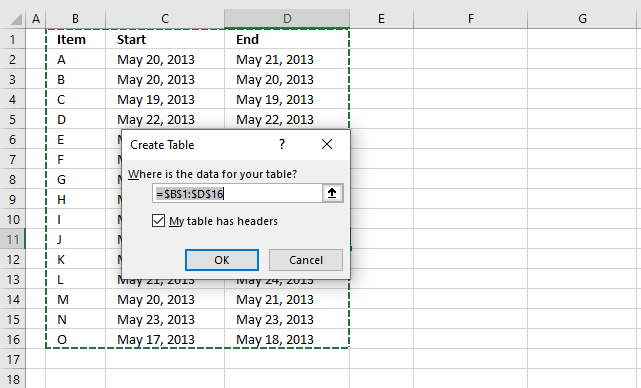An Excel Table has many great features, the one we are most interested in for today's tutorial is "structured references". It is basically a cell reference to an Excel Table, however, more advanced but still easy to construct.

This makes it possible to apply conditional formatting to all data in Excel Table even if data is added or deleted.

1. Select any cell in your data set.
2. Press short cut keys CTRL + T and "Create Table" dialog box appears, see image above.
4. Press with left mouse button on OK to apply settings and create Excel Table.

The cell formatting changes to indicate that the data set is now an Excel Table, you have the option to change the Table style if you want.

Go to tab "Table Design" on the ribbon, press with left mouse button on the button shown in the image below to expand the list of Table Styles.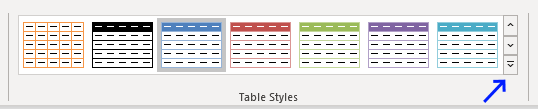Hover over a table style and your Excel Tables instantly changes to show what it looks like. This makes it easy to preview a table style and pick one that you like in no time.

## 2. Event code

The code below must be placed in a worksheet module to work properly, you cant put it in a regular module. This macro is triggered when the user selects any cell in columns C or D.

It copies the selected dates to cell range F2:G2 in order to highlight the appropriate dates using conditional formatting in the next step.

```'Event code
Private Sub Worksheet_SelectionChange(ByVal Target As Range)

'Check if selected cell is in column C or D
If Not Intersect(Target, Range("C:D")) Is Nothing Then

'Save dates from column C and D to cell range F2:G2
Range("F2:G2") = Range("C" & Target.Row & ":D" & Target.Row).Value
End If
End Sub

```

## 3. Where to put the event code?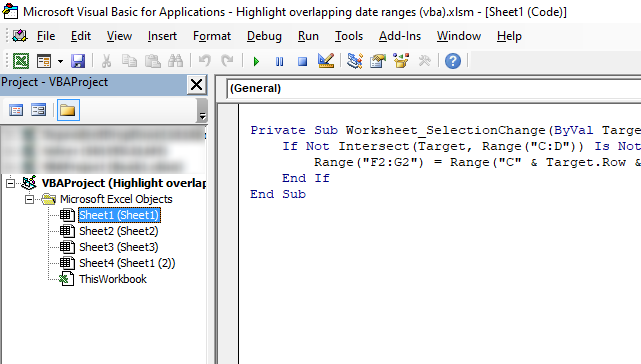The image above shows the Visual Basic Editor, it contains the project explorer to the left and a window to the right showing the VBA code if any.

1. Copy the above event code. (CTRL + c).
2. Press with right mouse button on on sheet name.
3. Press with left mouse button on "View code" and Visual Basic Editor appears with the corresponding worksheet module open.
4. Paste event code, see image above. (CTRL + v).
Note, save your workbook with file extension *.xlsm (macro-enabled workbook) to attach the code to the workbook.

## 4. Conditional formatting formula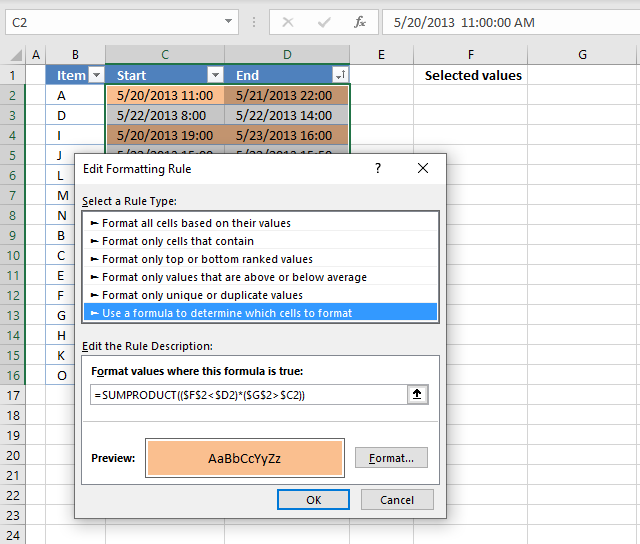1. Select date ranges in the Excel Table, the image above shows cell range C2:D16 selected.
2. Go to tab "Home" on the ribbon.
3. Press with left mouse button on the "Conditional formatting" button located on the ribbon.
4. Press with left mouse button on "New Rule..".
5. Select Rule Type: "Use a formula to determine which cells to format". See image above.
6. Enter the Conditional Formatting formula shown below these steps.
7. Press with left mouse button on the"Format..." button.
8. Press with left mouse button on tab "Fill".
9. Pick a fill color.
10. Press with left mouse button on Ok.
11. Press with left mouse button on OK

Conditional formatting formula

=(\$F\$2<\$D2)*(\$G\$2>\$C2)

### 4.1 Explaining CF formula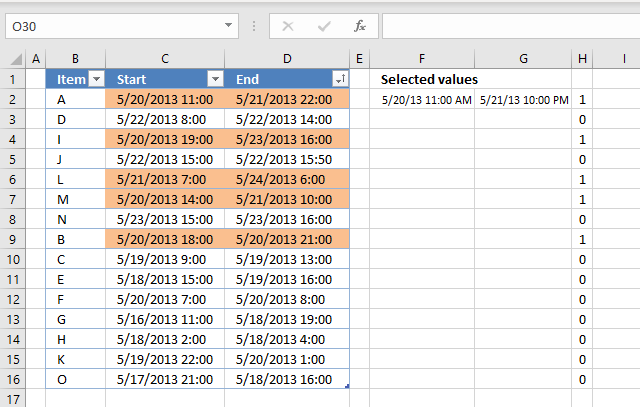I recommend that you enter the formula in cell H2 to easily see how the CF formula works. Copy cell H2 and paste to cell range H3:H16.

Excel has a built-in tool named "Evaluate Formula" that allows you to see formula calculations for the selected cell step by step.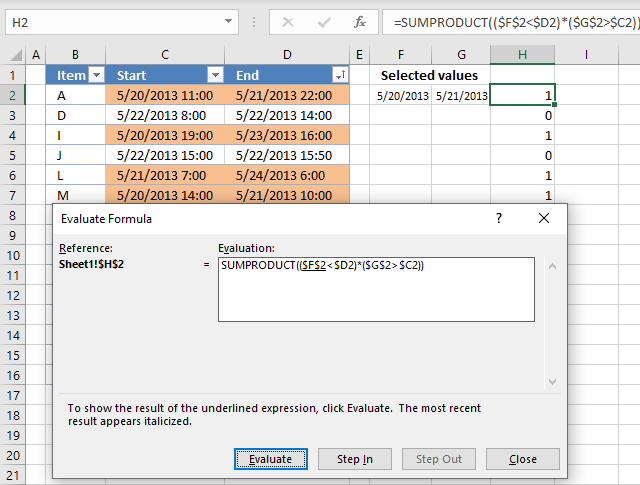Go to tab "Formulas" on the ribbon. Press with mouse on the "Evaluate Formula" button. A dialog box appears, see image above.

The "Evaluate" button takes you through the formula calculations step by step, the underlined part of the formula is what will be evaluated next time you press the Evaluate" button.

Italic text shows the result. Press with left mouse button on the "Evaluate" button repeatedly until you are pleased or the formula has been completely calculated. Press with left mouse button on "Close" button to dismiss the dialog box.

I have described each step in the formula calculation entered in cell H2 below. The formula corresponds to the CF formula applied to cell range C2:D2.

#### Step 1 - Check if date is smaller than selected date in cell F2

The less than sign checks if the date in cell F2 is smaller than the date in cell D2. The result is the boolean values True or False.

Excel handles dates as numbers, however, cell formatting shows them as dates. For example, enter 1 in any cell. Select the cell and press CTRL + 1 to open the "Format cells" dialog box.

Apply any date formatting, press with left mouse button on OK button. The cell now shows 1/1/1900, this proves to you that Excel handles dates as numbers. A date less than another date means it is a smaller number meaning it is earlier than the other date.

Time is the decimal part of a number. 1/24 is an hour.

Cell reference \$F\$2 is an absolute cell reference meaning it does not change when the cell is copied to cells below, this applies also to Conditional formatting formulas as well.

Cell reference \$D2 is only locked to the column and the row number is relative meaning it changes from cell to cell.

\$F\$2<\$D2

becomes

41414.4583333333<41415.9166666667

and returns boolean value True.

#### Step 2 - Check if date is larger than selected date in cell G2

\$G\$2>\$C2

becomes

41415.9166666667>41414.4583333333

and returns TRUE.

#### Step 3 - Multiply results

Multiplying boolean values is the same as applying AND-logic. True * True = 1, True * False = 0 and False * False = 0.

The numerical equivalent to True is 1 and False is 0 (zero).

The parentheses allow you to control the calculation, we want to evaluate the less than and larger than signs before we multiply the results.

(\$F\$2<\$D2)*(\$G\$2>\$C2)

becomes

TRUE * TRUE

and returns TRUE. Cell C2 and cell D2 will be highlighted by the Conditonal Formatting tool.

## 5. Sort highlighted values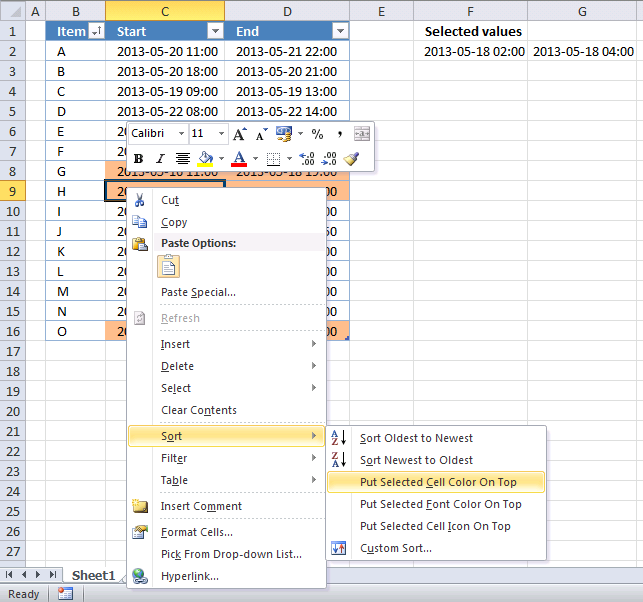Finding conditionally formatted values in a large Excel Table is not easy but there is a way that can be useful. This method sorts all conditionally formatted values at the top of the Excel Table.

1. Press with right mouse button on on a cell that is highlighted.
2. Press with mouse on Sort, see image above.
3. Press with mouse on "Put selected cell color on top".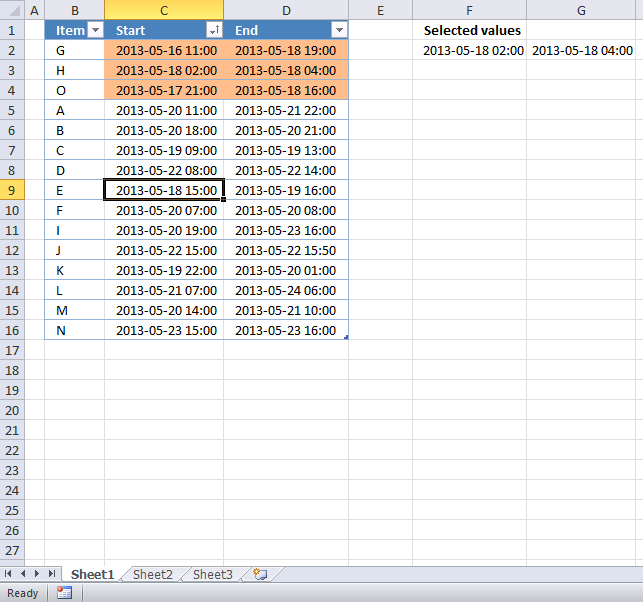## 6. Hide values in cell F2:G2You can hide the dates if you don't want the selected dates to be shown in cell range F2:G2. The following steps demonstrate how to format cells manually.

1. Select cell F2:G2
2. Press with right mouse button on on cells.
3. Press with left mouse button on "Format cells..."
4. Select category:  "Custom"
5. Type ;;;
6. Press with left mouse button on OK

They will hide values in cell range F2:G2, however, they still exist there. You can check that by selecting either cell F2 or G2 and the values show up in the formula bar.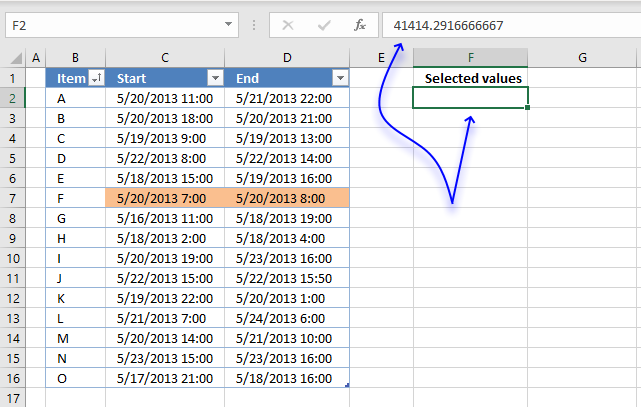The formula bar shows a number with decimals, this is how Excel handles dates. They are simply numbers formatted as dates, 0 (zero) is 1/1/1900 and 1/1/2000 is 36526.

Time values are decimal numbers. 12:00 A.M. is 0 (zero) and 1/24 is 01:00 A.M. 23/24 is 11:00 P.M.

Combine both date numbers and time numbers and you get 36526.5 equals 1/1/2000 12:00 P.M.